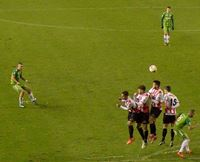# Relative Frequency

How often something happens divided by all outcomes.### Example: Your team has won 9 games from a total of 12 games played:

• the Frequency of winning is 9
• the Relative Frequency of winning is 9/12 = 75%

All the Relative Frequencies add up to 1 (except for any rounding error).### Example: Travel Survey

92 people were asked how they got to work:

• 35 used a car
• 42 took public transport
• 8 rode a bicycle
• 7 walked

The Relative Frequencies (to 2 decimal places) are:

• Car: 35/92 = 0.38
• Public Transport: 42/92 = 0.46
• Bicycle: 8/92 = 0.09
• Walking: 7/92 = 0.08

0.38+0.46+0.09+0.08 = 1.01

(It would be exactly 1 if we had used perfect accuracy)

Try it yourself:

images/rel-freq-user.js

1449, 1450, 1451, 1452, 3128, 3129, 3130, 3131, 3786, 3787